Learning Automata Based Channel Assignment with Power Control in Multi-Radio Multi-Channel Wireless Mesh Networks | OMICS International
Journal of Telecommunications System & Management
All submissions of the EM system will be redirected to Online Manuscript Submission System. Authors are requested to submit articles directly to Online Manuscript Submission System of respective journal.

# Learning Automata Based Channel Assignment with Power Control in Multi-Radio Multi-Channel Wireless Mesh Networks

Beheshtifard Z1* and Meybodi MR2

1Department of Computer Engineering and Information Technology, Islamic Azad University, Qazvin Branch, Qazvin, Iran

2Department of Computer Engineering, Amirkabir University of Technology, Tehran, Iran

*Corresponding Author:
Ziaeddin Beheshtifard
Department of Computer Engineering and Information Technology
Qazvin, Iran
Tel: 4515658145
E-mail: [email protected]

Received Date: August 01, 2016; Accepted Date: September 02, 2016; Published Date: September 14, 2016

Citation: Beheshtifard Z, Meybodi MR (2016) Learning Automata Based Channel Assignment with Power Control in Multi-Radio Multi-Channel Wireless Mesh Networks. J Telecommun Syst Manage 5:139. doi:10.4172/2167-0919.1000139

Copyright: © 2016 Beheshtifard Z, et al. This is an open-access article distributed under the terms of the Creative Commons Attribution License, which permits unrestricted use, distribution, and reproduction in any medium, provided the original author and source are credited.

Visit for more related articles at Journal of Telecommunications System & Management

#### Abstract

Wireless channel assignment is one of the major challenging issues in multi hop wireless mesh networks (WMN) when there is the need to design them in a distributed fashion, specifically for multi-radio multi-channel (MRMC) systems. In this work, a new learning automata based channel and power assignment scheme which adaptively improve network overall throughput by expecting network dynamics was proposed. First, a utility function which reflected the user’s preference for the signal to interference and noise ratio (SINR) was applied, and then the transmitter power. The distributed channel assignment and power control problem is formulated as a multiple payoff stochastic game of automata. In this game, each user evaluates a channel and power selection strategy by computing a utility value. This evaluation is performed using a stochastic iterative procedure. The utility function that potentially reflected a measure of satisfaction of every node was used by every node as an environmental response for the current selected strategy chosen by the nodes. According to dynamics of system, the proposed algorithm assigned channels and powers to radio interface such that it minimized interference in the neighborhood of a node. The stability of the system was analyzed via appropriate Lyapunov-like trajectory; it was shown that the stability and optimum point of the system converged.

#### Keywords

Wireless mesh network; Channel assignment; Power control; Learning automata

#### Introduction

A wireless meshes network (WMN) architecture according to  shows that a WMN consists of mesh routers and mesh clients. The mesh routers are generally stationary nodes and form a multihop wireless backbone (referred to as the backhaul tier) between the mesh clients and the internet gateways (a gateway is the node directly connected to the wired network). Each mesh router operates not only as a host but also as a router, forwarding packets on behalf of other nodes that may not be within direct wireless transmission range of their destinations. Additionally, some of mesh routers operate as gateways that interconnect wireless backbone to the wired internet backbone. On the other hand, mesh clients form the client tier. They are either stationary or mobile, and can form a client mesh network with each other and with mesh routers. The gateway and bridge functionalities in mesh routers enable the integration of WMNs with various existing wireless networks such as wireless sensor, cellular, wireless-fidelity (Wi- Fi) and worldwide interoperability, for microwave access (WiMAX).

Simultaneous communication on 802.11 radios may be lead to capacity degrading, when the operation is within the same interference range . Fortunately, the IEEE 802.11 specification in physical layer makes available multiple orthogonal channels. For example, the 802.11b/g standard provides three such channels while the 802.11a standards allow up to twelve non-overlapping frequency channels which could be used simultaneously within the neighborhood. Accordingly, to achieve high throughput, wireless mesh routers can be equipped with multiple radios and operate on multiple orthogonal frequency channels which arises to the advantage of a self-managing and high capacity wireless mesh network .

To the best of our knowledge, this work is the first study on joint multi-radio channel assignment and power control under the physical interference model that can be implemented in a distributed fashion. In this work, a game of automata based channel assignment and power control scheme which does not require any knowledge about network topology and configurations, and which could be implemented in a fully distributed fashion under a more realistic model of physical interference was developed and adapted. The rest of the paper is organized as follows; the related studies on the channel assignment problem are summarized in section 2. Sections 3 and 4 focus on the system model that consist of the stochastic learning method used in learning automata and propose a distributed multi radio channel assignment and power control based on leaning automata that gradually learns the stochastic behavior of network. Section 5 maps the network model to a game of multi-automata model and analyzes the stability of the proposed scheme. The final section provides some simulation result and conclusions.

#### Channel and Power Assignment Problem

A node in wireless mesh networks needs to share a common channel with its neighbors. Assigning more radios to the same channel results in the achievement of more connectivity. Obviously, the establishment of more virtual links with neighbors increases interference. Therefore, there exists a tradeoff between maximizing connectivity and minimizing interference . Increase in neighbors who use the same channel can increase interference. Therefore a scheme that creates balance between maximizing the connections and reduction of interference is required.

The restrictions encountered by a channel allocation algorithm are as follows: First, the total number of channels is fixed. Second, number of separate channels for each mesh router limits the number of radios that it has. Third, two nodes that require communicating with each other need to share a common channel. Forth, total traffic on a connection that passes from a common channel should not be higher than the nominal capacity of the channel . Another aspect of WMN deployment problems is energy consumption, especially when mesh routers operate under MRMC configuration, particularly when such application is intended to be used in WMN deployed in rural and remote communities where electric power supply are sorely unavailable and nodes must operate by limited battery power supply . Another issue is related to network connectivity when nodes transmitting with high power shorten the network lifetime and as a result causes failure in network connectivity. According to a survey of wireless mesh network conducted in , power management aims to control connectivity, interference, spectrum spatial reuse, and topology. While mesh routers require the power management to control connectivity, mesh clients may need some protocols to be efficient in power usage. Thus, it is quite possible that WMNs require power management to optimize both power efficiency and connectivity, which results in a complicated problem.

In some studies, channel assignment problem is modeled by graph coloring problem, but this approach is incapable of satisfying all of the restrictions earlier mentioned. For instance, standard graph coloring does not consider all the mentioned constraints [10,11]. Node multi-coloring is unable to resolve common channel constraint . On the other hand, edge coloring violates the second constraint, such that the number of assigned color to each node cannot exceed the number of radios contained by each node. Although constrained edge coloring scheme covers the first tree constraint, it cannot resolve traffic constraint.

In terms of learning method, the present work is a generalization of previous study based on stochastic channel assignment . In terms of channel assignment, this work is similar to the approach presented in  but different in channel state expectation. In , the authors proposed an approach that each mesh node discovered its neighbors and the channel usage in its neighborhood periodically. In the proposed scheme, nodes do not need to pass messages with neighbors, and independently expect channel state according to the ability of packet transmission.

In the context of multi-channel MAC protocols with power control, many works have been proposed [15,16]. The main idea behind their power control scheme is the adjustment of different power levels for data and control packets, such that control packets (CTS, RTS, and RES) will always be sent using maximum power Pmax because of their responsibility to warn neighboring environment of the future communication activity between the sender and receiver. However, data packets will always be used with the proper power control. But some experiments in  investigated the impact of interference caused by signal power leakage when the radios were in close proximity. Therefore the use of a proper power level for all control and data packets improves the ability of a node to reach its neighbor with the best channel qualities .

In recent studies, joint multi-radio channel assignment with power control and routing protocol were proposed in . The authors proposed a heuristic method called MP-CARA for the minimum power channel assignment and routing problem. MP-CARA is a centralized algorithm and requires full knowledge of the current network status, such that it requires a large amount of message passing between nodes when there is the need to be implemented in the real world.

This paper addresses the channel assignment problem and specifically investigates optimal channel and power assignment in wireless mesh networks using learning automata. In this approach, the nodes do not require any knowledge about network topology, and heuristically learn contention for better channel and power selection in a distributed fashion. The current algorithm intelligently selects channels and power level for the mesh radio in order to maximize desired utility function for each user. On the other hand, this algorithm tries to minimize ripple effect which decreases the throughput of the system because of channel oscillation.

#### System Model

This section presents an overview of learning automata as an approach for dynamic optimization for the stochastic behavior of wireless mesh networks. This unknown behavior affects some network conditions such as distortion and fading which are unknown in considering real-time applications. The online algorithms are implemented in real-time in order to cope with unknown application characteristics, network dynamics and resource constraints. This section also presents a problem formulation for the channel assignment problem.

Utility function

The utility function is defined as the level of satisfaction derived by a user from undertaking an activity. In the wireless data network, the utility function is related to the amount of information transmitted by the user in the lifetime of its battery. According to this definition, the utility function can be expressed as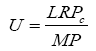(1)

Utility as defined before is the number of information bits received successfully per joule of energy expended. Where Pc denotes the probability of correct reception of a frame at the receiver. The frame success rate can be expressed as Pc=(1-Pe)M where Pe is bit error rate (BER) and M is the packet length in bits. Also L is the length of information of M bits at a rate R bit per second using P watts of power. If the SNIR is denoted by γ, according to modulation scheme BER can be expressed as a function of SINR (Table 1).

Modulation Scheme BER
BPSK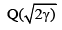DPSK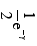Coherent FSK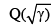Non Coherent FSK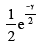Table 1: Bit error rate as a function of SINR for different modulation schemes.

According to efficiency function declared in  as f (γ)=(1-2Pe)M, the resulting utility function is given as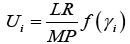bits/joule (2)

and γi (SINR of user i) is defined as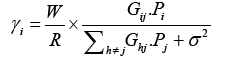(3)

and W is spread spectrum bandwidth in Hz, σ2 is additive white Gaussian noise (AWGN) power at the receiver and Gi j is the path gain of sender i on receiver j.

Learning automata

A learning automaton is an adaptive decision-making mechanism situated in a random environment that learns the optimal action through repeated interactions with its environment. This present discussion focuses on a specific type of learning automata called variable structure stochastic learning automata [21,22]. The stochastic automaton attempts a solution of the problem without any information on the optimal action (initially, equal probabilities are attached to all the actions). One action is selected at random, the response from the environment is observed, action probabilities are updated based on that response, and the procedure is repeated. A stochastic automaton acting as described to improve its performance is called a learning automaton.

A single learning automaton is suitable for learning the optimal action from a finite set of alternatives available. However, when the number of actions is large (even though finite), learning becomes slow. There are essentially two reasons for this. The initial action probabilities are generally set as 1/r, where r is the number of actions and these probabilities become small when r is large. A large number of iterations are thus needed for the best action probability to increase from 1/r to unity. The second reason is the computational overhead of the large number of probabilities updating needed at each instant. Also, with large number of actions, it is often the case that the reward probabilities are closer and hence it becomes difficult to distinguish the optimal action from others. This implies that there is the need to employ a smaller value of reward probabilities, thus resulting in a larger number of iterations for convergence.

We define the learning environment of the system constructed with a network of learning units say LU1, LU2,.…, LUn so that each LU corresponds to a multi-radio wireless node. Each LU is equipped with two automata for joint channel assignment and power control, respectively as shown in Figure 1. Formally, every learning unit can be described by 2-tuple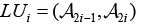such that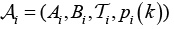where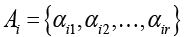is the finite set of actions,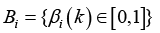is the set of possible input or reinforcement to the Ai as a random payoff, Ti is the learning algorithm for updating action probabilities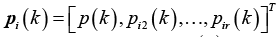is the action probability vector at time instant k, where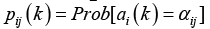and αij is the jth action of Ai.

Let βi be the payoff to the ith LU player, we define functions: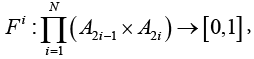by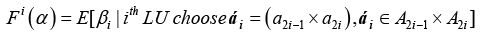Fi (α) is called the payoff function for player i. The player receives the payoff signal (βi) and they have no knowledge of other payoff functions.

Definition 1: The vectoris an optimal point of the game if for each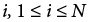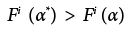for all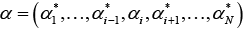such that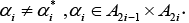Remark 1: In the aforementioned definition, the condition implies that α* is Nash equilibrium of the game matrix F (.) indexed by αi, 1 ≤ i ≤ N.

The operation of a LA is based on the probability updating algorithm, also known as the reinforcement scheme. This algorithm utilizes the environmental response which was received as a result of performing the action selected at cycle n (α (n)), in order to update the probability distribution vector p. After the updating is performed, the LA selects the action to perform at cycle n + 1, according to the updated probability distribution vector p (n + 1).

Let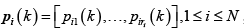denote the action probability distribution of ith automata, where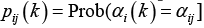and αij is the jth action of ith automata. At cycle k, ith automata select action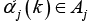according to the probability distribution pi (k). Then the lth learning unit (containing two automatareceives reinforcement βl (k) from the environment that is the response to the action tuple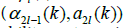. Finally, every automaton updates their action probability vector using the Linear Reward-Inaction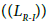algorithm as follows: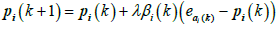(4)

Where eαi (k) a unit is vector of dimension ri with αi(k)-th element unity, and λ ? (0, 1) is the step size parameter of the algorithm.

#### Channel Assignment and Power Control Algorithm

In the multiple payoff game, each node acts as a player or learning unit which participates in channel assignment and power control game. According to defined utility function as a satisfaction factor of the player, the objective of each player is to maximize its payoff which can be measured by its utility function. The only information that is available for the player is its payoff after each play. Each learning unit as a player does not have any knowledge about the strategies used by other players and also the environment response for possible play. Therefore in non-cooperative channel assignment and power control game, each player (node) maximizes its utility in a fully distributed fashion which can be expressed as: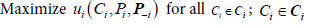The solution of this problem gives Nash equilibrium so that in Nash equilibrium, given the assigned channels and the power levels of other player, no player can improve its utility level by making individual changes in selected channels and power level.

Definition 2: A resource vector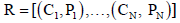is a Nash equilibrium of the non-cooperative game if, for every i∈ N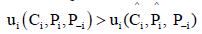for all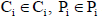Channel assignment and power control in multi-radio wireless mesh networks involves allocating a channel for each mesh radio interface and adjusting the suitable power level in order to achieve better channel utilization and decrease interference between channels. In other words we intend to maximize utility of each user with better usage of resources such as power, channel and radio interfaces.

A distributed learning automaton based algorithm that dynamically adapts nodes channel selection and power level according to measured payoff is proposed. Each node is equipped with two learning automata that takes an action for every automaton on each time slot T. The first automaton is used for channel assignment, and its action set corresponds to the subset of available channels constructed by a combination of (Mi (number of available radios for node i) from the number of available channels (K). The second automaton is used for power level control, and its action set corresponds to the finite set of discrete power level. The quantization of the power level is based on power level in digital cellular systems like GSM such that the uplink and downlink transmission power are discretized and may vary from 5 to 33 dBm at values equally spaced by 2 dBm.

Algorithm 1: Distributed channel assignment and power control (LA-DCAPC)

Step 1: Parameters definition

1. δ: Threshold for terminating algorithm

2. K: Number of available channels

3. Mi : Number of available radios for node i

4. A2i-1: Action set for channel assignment automaton in LUi

5. A2i: Action set for power level control automaton in LUi

Step 2: Initialization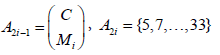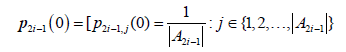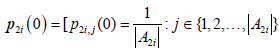Step 3: Search

1. Repeat for timeslot k=1, 2, ……

Pick an action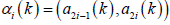according to its action probability vector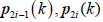Each player assigns the corresponding channel set to its radios, although adjust the power level based on selected action on its second automation.

Each player obtains a payoff ui (k) on the all of selected actions, and then normalize ui as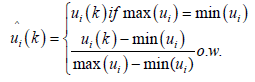(5)

Each player updates its action probability vector for automata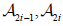according to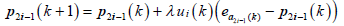(6)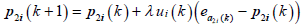(7)

If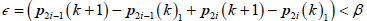stop the algorithm ; else go to step 3.1.

The minimum and maximum values of utility function are determined dynamically for normalizing the utility function to lie in the interval (0, 1), but the realistic value for the maximum and minimum cannot be determined in advance. In other words, the current max (ui), min (ui) is approximation values for actual maximum and minimum. Alternatively, a zero can be chosen for min (ui) and a large constant value for max (ui), but choosing a very large value for maximum compared to average utility values will significantly decrease the speed of convergence.

In this scheme, each node tries to maximize its utility function by maximizing the normalized payoff via current assigned channels and power level. Nodes adaptively learn which channel-set and power level are the best to improve their utility function. According to linear reward-inaction scheme (LR-I) reflected in the update equation??, when a node picks a channel set and power level as its actions, and when a chosen channel set and power level results in a reward, then the probability of choosing that action in the next time slot is updated, if not, no change takes place.

Analysis

This section analyzes the stability of learning method in the game of multi-automata. A play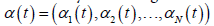of n automata is a set of strategies chosen by the automata at stage t, such that αi (t) is the action selected by ith automata. Also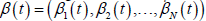is the payoff vector such that βi (t) is the payoff to ith automata.

Let the action set of all automata denoted by A with |A|=r such that r is the number of combinations that can be assigned to the channels to available radios in a node.

Define function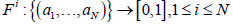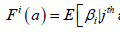automata chose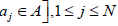(8)

Fi is the payoff function for player i. The players only receive the payoff, such that they have no knowledge of these functions.

Two known theorems used for the stability of systems are stated as follows:

Theorem 1: Consider the discrete-time system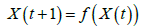Where X is a vector, f is a vector such that f (0)=0. Suppose there exists scalar function V(X) continuous in X such that: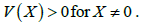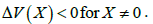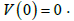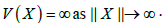Then equilibrium state X=0 is asymptotically stable and V(X) is a Lyapunov function.

Theorem 2: if the function f(X) is defined as: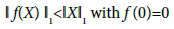(10)

for some set of X ≠ 0 the aforementioned system is asymptotically stable and one of its Lyapunov function is: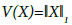(11)

These two theorems and their proofs are given in .

Theorem 3: A multi-player game of automata using the proposed learning scheme in a stationary environment reaches the pure optimal strategy.

An Identical analysis for single automaton was proposed in . In this study it was easily shown that the 1st norm of expected value of all sub-optimal action converges to zero. For example, for ith automaton if α1 is optimal action and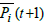is a vector of expected value for other sub optimal action in steady state, it can be shown that: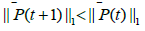(12)

It is a known fact that every automaton in the network chooses an action independently based on its action probability vector, so this analysis can be extended to the matrix of all probability vectors. So if we define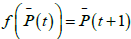and extending P (t) to matrix of all probability vectors of every automaton, then it has been proven that, the expected values of the probabilities of the sub-optimal actions all converge to zero. This implies that the probability of the optimal action converges to 1, and according to Lyapunov function the stability of the optimal point is proven .

#### Experimental Results

In this section, the performance of the learning automata based channel assignment and power control scheme was evaluated through detailed numerical studies. A grid wireless multi hop mesh network topology was generated using the following method. A square region was first specified in the area of 2500 × 2500 meter-square which has the width [0, 2500] on the x axis and the height [0, 2500] on the y axis. Then a 6×6 number of nodes was generated and placed at the same distance from each other. Rayleigh fading was used for signal fading model and free space path loss model for the loss in signal strength. All the mesh routers were fixed and only client nodes were mobile. Default radio frequency, radio reception sensitivity and radio reception threshold were subsequently assumed as 2.4 GHz, -91 dBm and -81 dBm, and also for simplicity ambient noise was fixed to -50 dBm. Sensing and receiving modes were determined based on physical situations of radio signal propagation.

The network topology used in the present experiment was a grid style as depicted in Figure 2. Each node was equipped with two learning automata which evaluated the response of the environment, according to measured utility as defined by equation 1. The first learning automaton of each node randomly selected R channel from K=10 orthogonal channels and the second automata chooses the random power level from available discrete power level set in the first time slot. After channel allocation and power adjusting, nodes began sending and receiving packets according to the defined traffic flows. At the end of the every time slot, normalized utility as environment payoff was measured by the nodes. At the beginning of each time slot, nodes evaluated the environment response to last channel-set allocation and power level adjusting and rewarded/penalized their automata by updating action probabilities.

In the first scenario it was assumed that there were four concurrent flows such that F1 from node (1,5) to (5,5), F2 from (2,4) to (3,5), F3 from (5,3) to (1,2) and F4 from (4,1) to (5,1). First, the evolution of the average length of the queues and throughput for each flow was studied. The simulation results, as shown in the Figures 3 and 4, indicate the total number of packets and throughput for each flow tends to a constant value in steady state. The first result of observation was that the throughputs of the flows converged to completely different values according to the separation of the source and destination. For example the longest hop flow F1 achieved the lowest throughput versus F4 which reached the highest throughput with only 1 hops length. Total network dynamics in long time period showed that system can efficiently adapt to their behavior according to environment response. Despite the minor oscillations in delivered packets in short time periods, the network can efficiently learn how to choose appropriate channels that deliver more packets with low interference and more connectivity.

In the second scenario, the performance of DCA-PC algorithm and learning automata based channel and power assignment scheme that gradually learns truly decision on channel and power level selection were compared. The performance of the algorithm is illustrated in Figure 5. It showed that the total backlog changed quickly and converged to a steady state value as compared to the DCA-PC algorithm. Although the total backlog value in the steady state was significantly lower than the DCA-PC scheme, suggesting that in comparison with this scheme, the greater number of packets to the destination was reached. This was due to the full utilization of all physical radio connections against separation of resource to control and data messages in algorithm against DCA-PC. Figure 6 showed that a similar result was obtained when throughput was studied among two discussed schemes. It clearly showed that the throughput of the proposed method was significantly higher than the DCA-PC.

Finally, the performance of the algorithm was investigated in aspect of power consumption. The random topology placed in a square region with the area of 10000×10000 meter-square was used, 16 wireless nodes were spread in this area randomly as depicted in Figure 7. Figure 8 shows the simulation results of the power consumption, adaptation of selected nodes according to their position and distance from other nodes. For example, node 12 was of little distance from its neighbors and it gradually learned how to adapt its transmission power to achieve maximum utility with the passing of time slots. On the other hand, nodes 3 and 8 were located relatively far away from each other. So they learned to use maximum power to achieve the intended throughput.

#### Conclusions

In this paper, learning automata based distributed and online channel assignment with power control for multi-radio; multi-channel wireless mesh networks were proposed. According to unknown characteristics of wireless mesh networks, mesh routers equipped with learning automata can predict appropriate utility function with environment response of the networks for last behavior of network components. This distributed fashion of online channels and power allocation permits the network to achieve an optimum point of overall network throughput. This mechanism was analyzed by expecting the sub-optimal action probabilities, such that stability theory was used to characterize the dynamic behavior of the proposed approach. In addition, with Lyapunov-like function, the stability and optimum point converged under some mild restriction. The experimental results showed that the proposed scheme provides significant improvement in network behavior and can establish good balance for network connectivity and power saving.

#### References

Select your language of interest to view the total content in your interested language

### Article Usage

• Total views: 9476
• [From(publication date):
December-2016 - Nov 22, 2019]
• Breakdown by view type
• HTML page views : 9291Can't read the image? click here to refresh